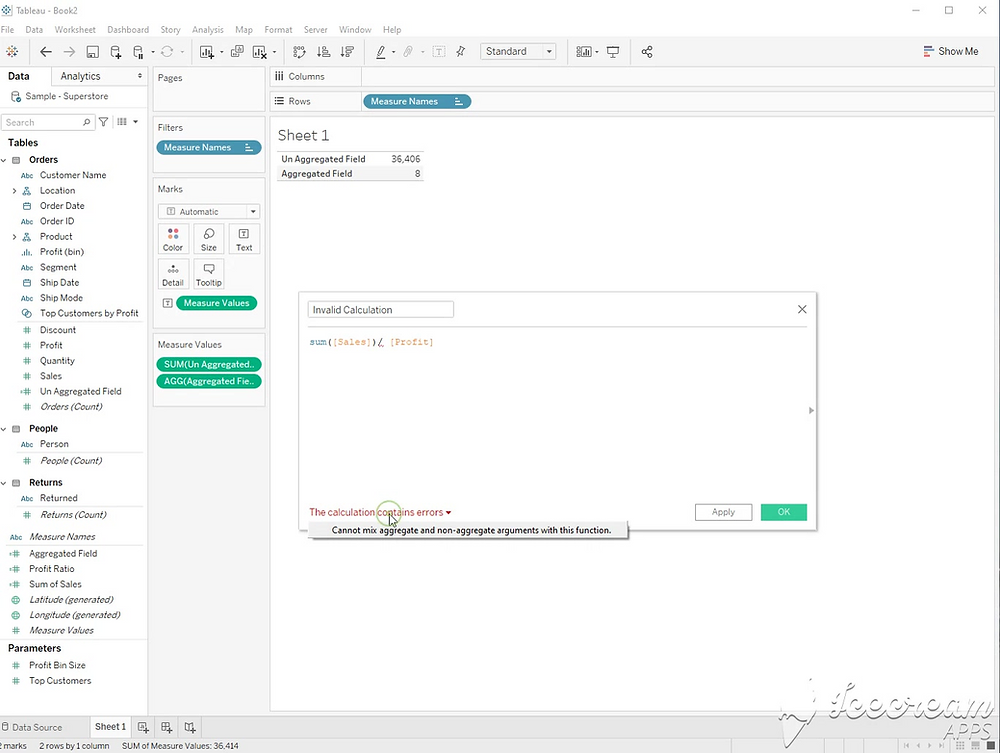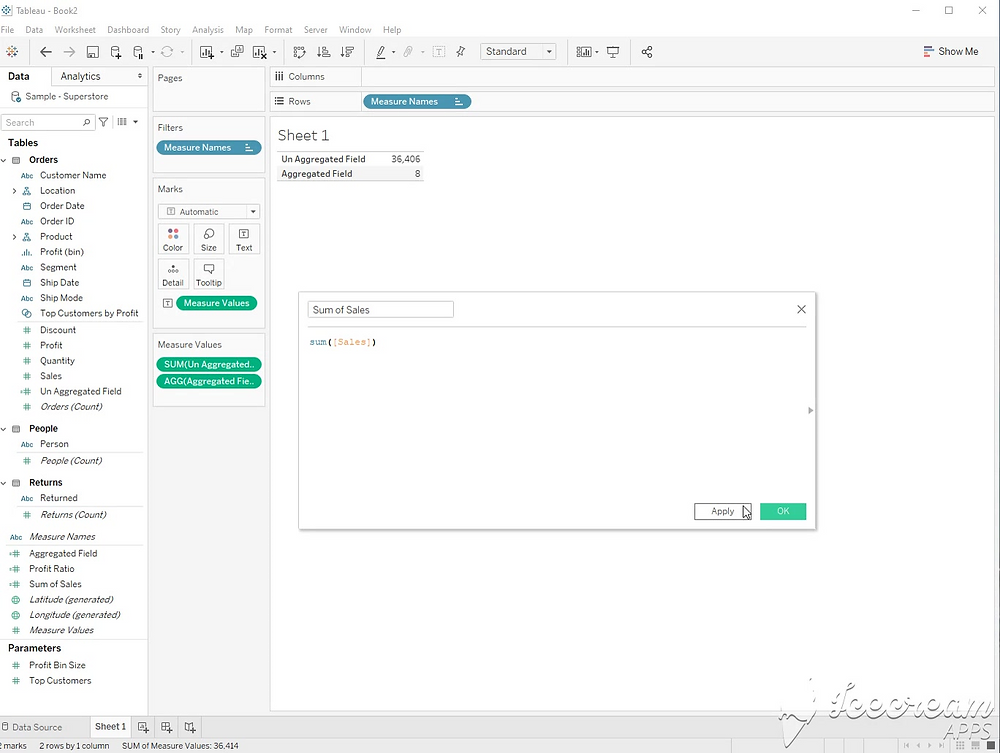Search
• Robert Breen

# Tableau: How to Solve “Cannot Mix Aggregate and Non-Aggregate” Errors

Updated: May 14Let’s start with a simple example. Within a single calculation, all parts of a calculation must be either aggregated or not aggregated.

This calculation is not aggregated.

[Sales] / [Profit]This calculation is aggregated.

Sum([Sales])/sum([Profit])Both of the calculations are valid. This calculation includes both aggregate, and non aggregated fields.

Sum([Sales]) / [Profit]

This calculation is not valid.It gets more complex when using other calculated fields in a second calculation. In this example, I created a field called “Sum of Sales” with this calculation. Sum([Sales]).If I use that field later, all other fields in the calculation must also be aggregated.

This calculation will be valid.

[Sum of Sales] / Sum([Profit])

Since [Sum of Sales] was already aggregated in a previous calculation, [profit] will also need an aggregation.A few notes:

- Common aggregations include SUM, AVG, COUNT, MIN, MAX

- All Table calculations are aggregated

- Level of detail calculations using FIXED, INCLUDE, and EXCLUDE are not aggregated.